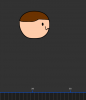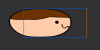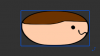# Legacy GMRotate with an offset, without changing sprites X&Y offsets

#### Liam Jacobs

##### Member
Hi all,

I'm a bit stumped on this one at the moment. I'm trying to set up a system where a sprite can be rotated as if it had a specific x and y offset, without the sprite actually having the offset.

Here's why:

I've written a bit of code that allows the user to drag the mouse around in order to scale a sprite. The drawing code I'm using is as follows:

Code:
``draw_sprite_ext(spr, 0, x, y, w / sprite_get_width(spr), h / sprite_get_height(spr), ang, c_white, opc);``
Pretty simple stuff. I'm changing the X and Y scale to the 'w' and 'h' variables (the users selected width and height) and then dividing that by the sprite's original width/height.

The problem arises when the sprite has an offset. The sprite is scaled based on that offset. In other words, if I want to scale a sprite so that it's stretched to the right-hand side, or the left-hand side, the offset causes the sprite to be scale in either direction.

Does anyone know of a way to rotate the sprite as if it had a specific set of origin points?

For example, let's say we have a 100x100 sprite, with the origin point set to 0, 0. Is there any way to rotate that sprite as if the origin point were set to 50, 50?

Thanks kindly for your help.

#### flyingsaucerinvasion

##### Member

var _c = dcos(angle);
var _s = dsin(angle)
var _x = x - x_offset * _c - y_offset * _s;
var _y = y - x_offset * -_s - y_offset * _c;

#### Liam Jacobs

##### Member

var _c = dcos(angle);
var _s = dsin(angle)
var _x = x - x_offset * _c - y_offset * _s;
var _y = y - x_offset * -_s - y_offset * _c;

Hi! Thanks for the helpful reply. This works perfectly, just as expected. Just in case of some possible reworking of the code, is there any way to do the 'opposite' of this? For example, scaling the sprite in the desired direction without taking the offsets into account? Thanks a bunch, as per usual!

#### flyingsaucerinvasion

##### Member
What do you mean exactly?

#### Liam Jacobs

##### Member
What do you mean exactly?
My goal is to allow users to click on any one of the 4 sides of the sprite, and drag in/outwards to resize the sprite. Very similarly to how an image editing tool would.
Say, for example, we click on the right-hand side of the sprite and drag to the right (in order to 'expand' the sprite in that direction). To resize the sprite, we change the 'w' and 'h' variables, which are used when drawing the sprite in the following code:

Code:
``draw_sprite_ext(spr, 0, x, y, w / sprite_get_width(spr), h / sprite_get_height(spr), ang, c_white, opc);``
The problem is that if the sprite we're resizing has an X offset, the sprite will also scale to the left, instead of just the right.

Here's a few images:

Before scaling:After scaling the sprite (if it has an offset):After scaling the sprite (if it does not have an offset):My goal is to be able to scale the sprite so that it can stretched in a specific direction, instead of the scaling being based off of the origin points.

#### flyingsaucerinvasion

##### Member
What you're going to have to do is move the instance.

Code:
``````    //if stretching left
var _xd = x_scale_change * ( sprite_get_xoffset(sprite_index) - sprite_get_width(sprite_index) );
//if stretching right
var _xd = x_scale_change * sprite_get_xoffset(sprite_index);

//if stretching up
var _yd = y_scale_change * ( sprite_get_yoffset(sprite_index) - sprite_get_height(sprite_index) );
//if stretching down
var _yd = y_scale_change * sprite_get_yoffset(sprite_index);

x += _xd *  dcos(image_angle) + _yd * dsin(image_angle);
y += _xd * -dsin(image_angle) + _yd * dcos(image_angle);``````
Make sure you choose the correct amount for _xd and _yd based on which side you are stretching.

EDIT: Corrected above sentense, it isn't which direction you are stretching that changes how you compute _xd and _yd, it is which side you are stretching.

*_scale_change would be the amount that the indicated axis scale has changed. I.e., (image_xscale - old_image_xscale).

--------------------

EDIT_2: I just rememberd you were using your own sprite offset variable, which brings up two points.

1), you'll want to substitute your offset variables for sprite_get_xoffset/yoffset above. And these variables should be the position of the offset when the sprite is NOT scaled.

2) A correction needs to be made to my first response to your thread. You need to scale the offset variables by the current image scales:

var _x = x - off_x*image_xscale * _c - off_y*image_yscale * _s;
var _y = y - off_x*image_xscale * -_s - off_y*image_yscale * _c;

Last edited: